Question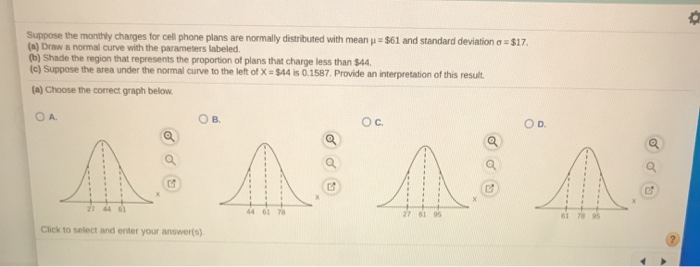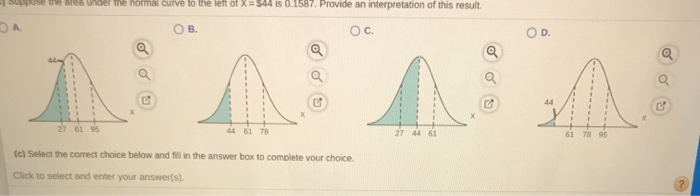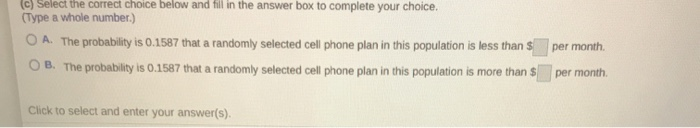(a)

The graph in option B is correct

(b)

The graph in option B is correct

(c)

The probability is 0.1587 that a randomly selected cell phone plan in this population is less than \$44 per month

#### Earn Coins

Coins can be redeemed for fabulous gifts.

Similar Homework Help Questions
• ### Suppose the monthly charges for ool phone plans are normaly distributed with mean μ-\$66 and stand...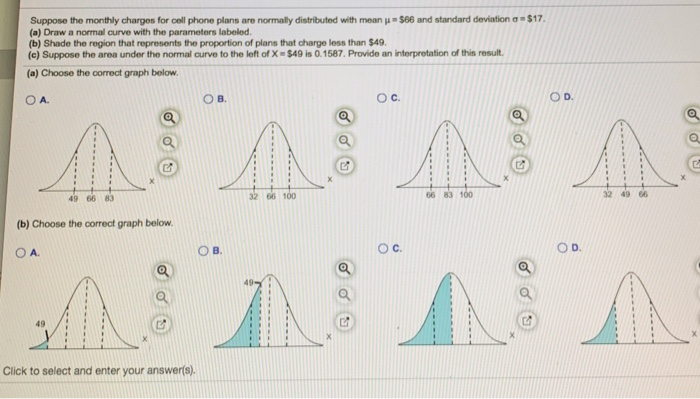please answer all parts Suppose the monthly charges for ool phone plans are normaly distributed with mean μ-\$66 and standard deviation at\$17 (a) Draw a normal curve with the parameters labeled (b) Shade the region that represents the proportion of plans that charge less than \$49 (c) Suppose the area under the normal curve to the left of X \$49 is 0.1587. Provide an interpretation of this result. (a) Choose the correct graph below ов. O C. O D. 49...

• ### This Question: 6 pts 13 of 15 (8 complete This Quiz: E QUE \$17. Suppose the...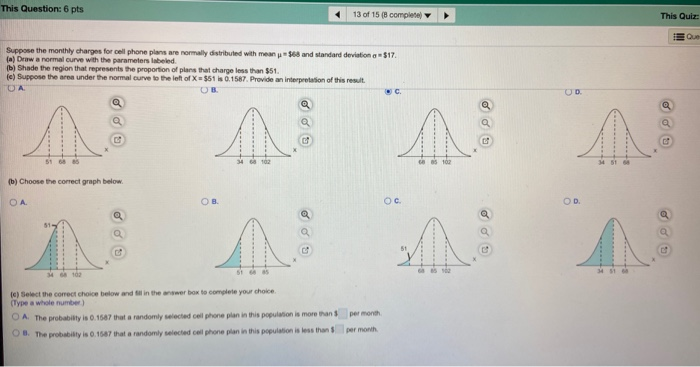This Question: 6 pts 13 of 15 (8 complete This Quiz: E QUE \$17. Suppose the monthly charges for cell phone plans are normally distributed with mean 3 68 and standard deviation (a) Draw a normal curve with the parameters labeled b) Shade the region that represents the proportion of plans that charge less than \$51. le) Suppose the area under the normal curve to the left of X = \$51 is 0.1587. Provide an interpretation of this result (b)...

• ### Applying the Concepts NW 31. You Explain It! Cell Phone Rates Monthly charges for cell phone...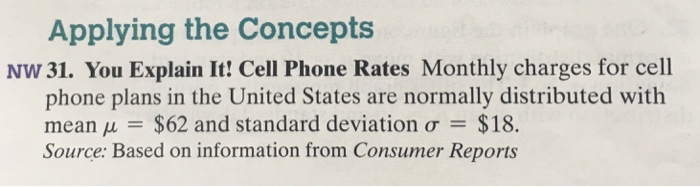Applying the Concepts NW 31. You Explain It! Cell Phone Rates Monthly charges for cell phone plans in the United States are normally distributed with mean μ \$62 and standard deviation σ \$18. Source: Based on information from Consumer Reports (a) Draw a normal curve with the parameters la (b) Shade the region that represents the proportion of plans beled. charge less than \$44 (c) Suppose the area under the normal curve to the left of x = \$44 is...

• ### 10 of 32 16 complete Suppose the birth weights of full-term babies are normally distributed with...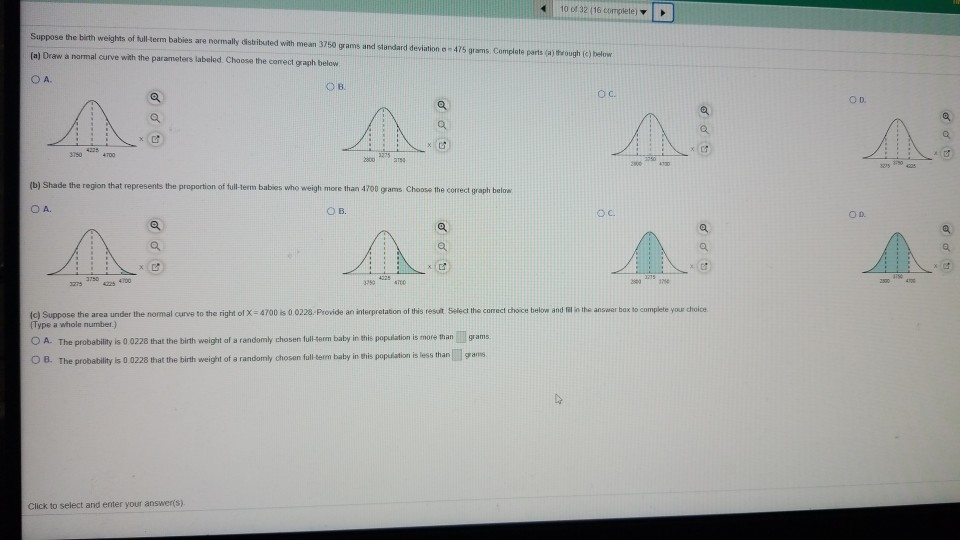10 of 32 16 complete Suppose the birth weights of full-term babies are normally distributed with mean 3750 grams and standard deviation - 475 grams Complete parts (a) through (c) below (a) Draw a normal curve with the parameters labeled. Choose the correct graph below ОА. OB OC OD no a 3150 4335 4700 3975 800 2000 4730 (b) Shade the region that represents the proportion of full-term babies who weigh more than 4700 grams Choose the correct graph below...

• ### o a Suppose the lengths of human pregnancies are normally distributed with p = 266 days...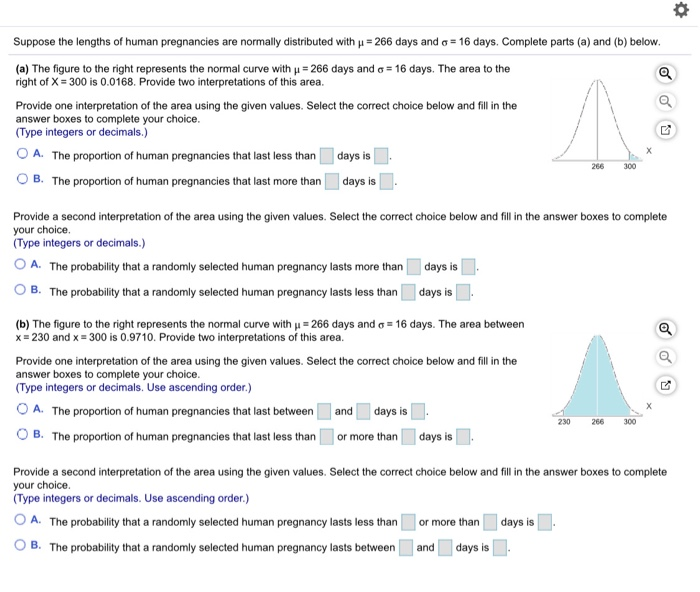o a Suppose the lengths of human pregnancies are normally distributed with p = 266 days and 6 = 16 days. Complete parts (a) and (b) below. (a) The figure to the right represents the normal curve with u = 266 days and o = 16 days. The area to the right of X = 300 is 0.0168. Provide two interpretations of this area. Provide one interpretation of the area using the given values. Select the correct choice below and...

• ### Suppose the lengths of human pregnancies are normally distributed with 266 days and a = 16...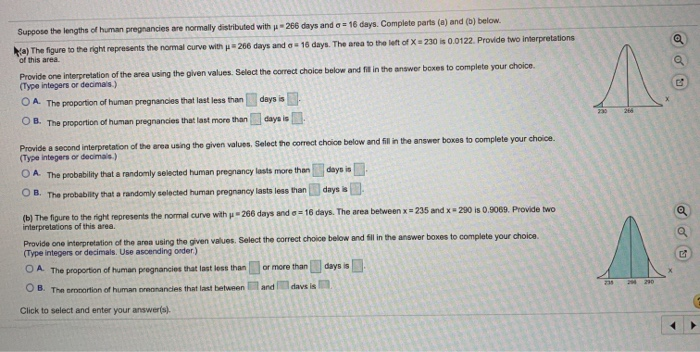Suppose the lengths of human pregnancies are normally distributed with 266 days and a = 16 days. Complete parts (a) and (b) below. a) The figure to the right represents the normal curve with 266 days and = 16 days. The area to the left of X = 230 is 0.0122. Provide two interpretations of this area Provide one interpretation of the area using the given values. Select the correct choice below and fill in the answer boxes to complete...

• ### i need help now please i dont get it. !!! Question Help o Steel rods are...i need help now please i dont get it. !!! Question Help o Steel rods are manufactured with a mean length of 25 centimeter (cm). Because of variability in the manufacturing process, the lengths of the rods are approximately normally distributed with a standard deviation of 0.07 cm. Complete parts (a) to (d) (a) What proportion of rods has a length less than 24.9 cm? (Round to four decimal places as needed.) (b) Any rods that are shorter than 24...

• ### Question Help -uppose the lengths of human pregnancies are normally distributed with u = 266 days...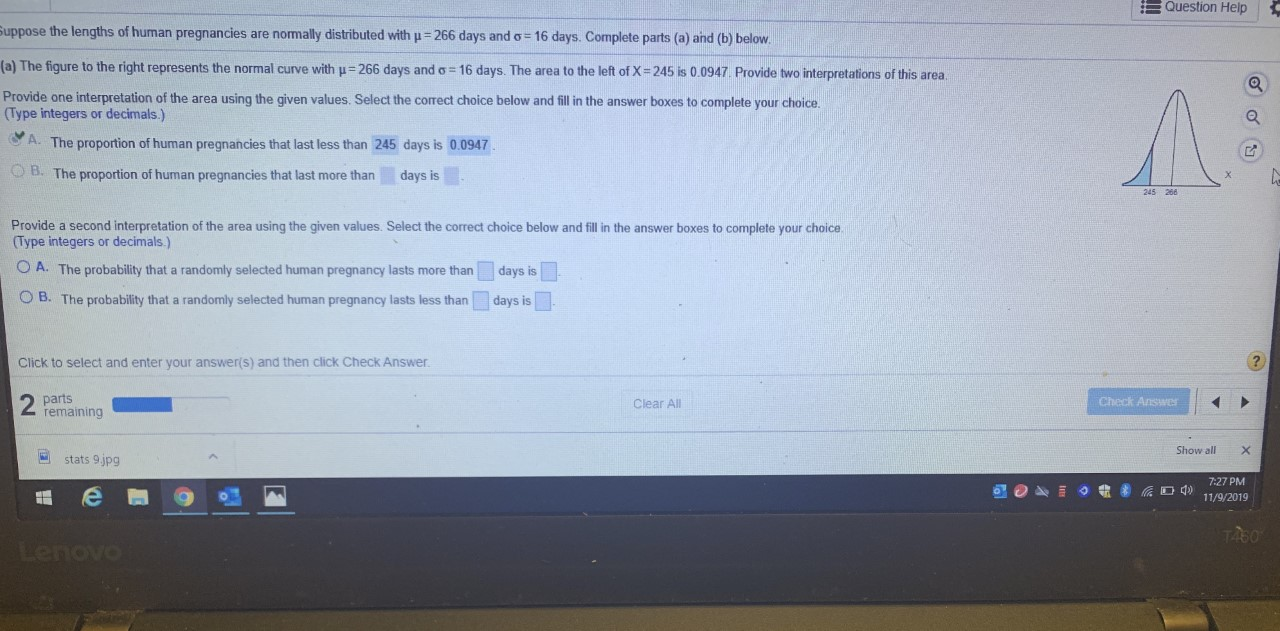Question Help -uppose the lengths of human pregnancies are normally distributed with u = 266 days and 6 = 16 days. Complete parts (a) and (b) below. (a) The figure to the right represents the normal curve with u = 266 days and o = 16 days. The area to the left of X = 245 is 0.0947. Provide two interpretations of this area Provide one interpretation of the area using the given values. Select the correct choice below and...

• ### Suppose the birth weights of​ full-term babies are normally distributed with mean 3600 grams and standard...Suppose the birth weights of​ full-term babies are normally distributed with mean 3600 grams and standard deviation σ = 480 grams. Complete parts​ (a) through​ (c) below. Suppose the birth weights of full-term babies are normally distributed with mean 3600 grams and standard de ation σ=480 grams. Complete parts a through c) below. (a) Draw a normal curve with the parameters labeled. Choose the corect graph below O A. C. Ο D. 3120/4080 28404560 264013600 3600 3600 3120 (b) Shade...

• ### Suppose the legis of human pregnancies are normally distribuled with u-286 days and o- 16 days....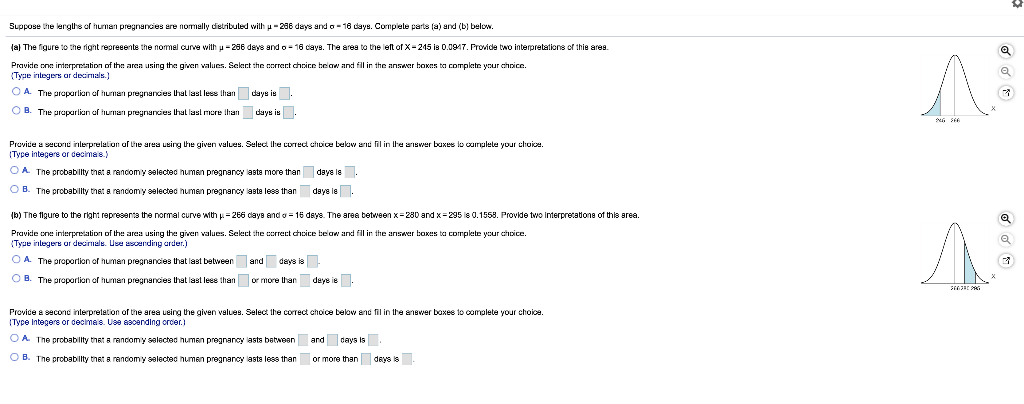Suppose the legis of human pregnancies are normally distribuled with u-286 days and o- 16 days. Cornplele parts (a) and (b) below. lal The figure to the right regreeente the normal curve with y - 286 days and o- 16 days. The area to the left of X - 245 is 0.09.7. Provide two interpretations of this ares. Pravide one interpretation of the area using the given values. Select the correct choice beow and fill in the answer boxes to...